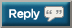# Thread: Help with rudimentary python code (reps)

1. ## Help with rudimentary python code (reps)

The prompt is:

Write a program to prompt the user for hours and rate per hours using input to compute gross pay. Pay the hourly rate for the hours up to 40 and 1.5 times the hourly rate for all hours worked above 40 hours. Use 45 hours and a rate of 10.50 per hour to test the program. ( The pay should be 498.75)

My code is:

hrs = input('Enter Hours: ')
rte = input('Enter Rate: ')
fhrs = float(hrs)
frte = float(rte)
if fhrs <= 40 :
pr = fhrs * frte
print(pr)
elif fhrs > 40 :
pr = (((fhrs - 40) * (1.5 * 10.50)) + (fhrs * frte))
print(pr)

My output is 551.25 and the desired output is 498.75

I have the correct spacing below the "if" and "elif" but for some reason my spaces aren't showing up when I post.2. while(true):
print("op is a phaggot")3. Change your hourly rate (X) to where pr = 498.75 in the equation pr = (((fhrs - 40) * (1.5 * X)) + (fhrs * frte))4. pr = (((fhrs - 40) * (1.5 * 10.50)) + (fhrs * frte))

The last portion of the equation is essentially adding the TOTAL hours worked (45 in this case) by the regular rate, instead of the base 40.

The input would be (((45-40)*(1.5*10.5))+(45*10.5))

Hopefully that guides you in the right direction5. Your coding sytnax is fine but your math/logic is wrong. You want only the overtime hours to get 1.5x the rate, not the entire duration.

Solution:
Spoiler!6. It is possible to create a DMARC checker with python ?#### Posting Permissions

• You may not post new threads
• You may not post replies
• You may not post attachments
• You may not edit your posts